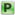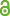Hauptmenü
• Autor
• Of, Günther
• Wendland, Wolfgang L.
• Zorii, Natalia
• TitelOn the Numerical Solution of Minimal Energy Problems
• Datei
• DOI10.1080/17476930903394986
• Erschienen inComplex Variables and Elliptic Equations
• Band55
• Erscheinungsjahr2010
• Heft11
• Seiten991-1012
• LicenceCC-BY
• Zugriffsrechte• Download Statistik612
• Peer ReviewJa
• AbstractWe study the constructive and numerical solution of minimizing the energy for the Gauss variational problem involving the Newtonian potential. As a special case, we also treat the corresponding condenser problem. These problems are considered for two two–dimensional compact, disjoint Lipschitz manifolds Γ_j ⊂ ℝ^3 , j = 1, 2, charged with measures of opposite sign. Since this minimizing problem over an affine cone of Borel measures with finite Newtonian energy can also be formulated as the minimum problem over an affine cone of surface distributions belonging to 1 the Sobolev–Slobodetski space H^−(1/2) (Γ) , Γ := Γ 1 ∪ Γ 2 , which allows the application of simple layer boundary integral operators on Γ, a penalty approximation for the Gauss variational problem can be used. The numerical approximation is based on a Galerkin–Bubnov discretization with piecewise constant boundary elements. To the discretized problem, the projection–iteration is applied where the matrix times vector operations are executed with the fast multipole method. For the condenser problem, we solve the dual problem which reduces in our case to solving two linear boundary integral equations. Here the fast multipole method provides an efficient solution algorithm. We finally present some convergence studies and error estimates.